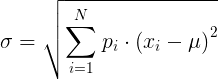# Standard Deviation Calculator

Standard deviation (σ) calculator with mean value & variance online.

## Population and sampled standard deviation calculator

Enter data values delimited with commas (e.g: 3,2,9,4) or spaces (e.g: 3 2 9 4) and press the Calculate button.

 Enter data values: Population standard deviation: Sample standard deviation: Population variance: Sample variance: Mean:

## Discrete random variable standard deviation calculator

Enter probability or weight and data number in each row:

Probability Data number
 Standard deviation: Variance: Mean:

## Whole population standard deviation calculation

Population mean: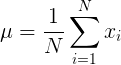Population standard deviation: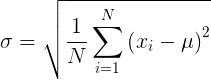## Sampled data standard deviation calculation

Sample mean: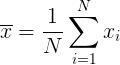Sample standard deviation: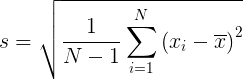## Discrete random variable standard deviation calculation

Random variable mean: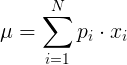Discrete random variable standard deviation: